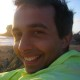author Arun PersaudSun, 18 Jan 2009 04:39:05 +0000 (20:39 -0800) committer Arun PersaudSun, 18 Jan 2009 04:39:05 +0000 (20:39 -0800)
every time a call is made (e.g. re/contra) a comment is added. So players can check when the call was made later

Fixes issue #12.

 include/game.php patch | blob | history

@@ -34,6 +34,7 @@ \$myname   = DB_get_name('hash',\$me);
\$mystatus = DB_get_status_by_hash(\$me);
\$mypos    = DB_get_pos_by_hash(\$me);
\$myhand   = DB_get_handid('hash',\$me);
+\$myparty  = DB_get_party_by_hash(\$me);
\$session  = DB_get_session_by_gameid(\$gameid);

/* get prefs and save them in a variable*/
@@ -119,17 +120,50 @@ if( \$mystatus != 'gameover' ||
\$myid == \$_SESSION['id']))
output_user_notes(\$myid,\$gameid,\$mystatus);

-/* handle calls */
+/* handle calls, output a comment to show when the call was made */
+\$comment  =  '';
+
+/* check for calls, set comment */
if(myisset('call')  && \$_REQUEST['call']  == '120' && can_call(120,\$me))
-  \$result = DB_query("UPDATE Hand SET point_call='120' WHERE hash='\$me' ");
+  {
+    \$result = DB_query("UPDATE Hand SET point_call='120' WHERE hash='\$me' ");
+    if(\$myparty=='re')
+      \$comment .= "Re";
+    else if(\$myparty=='contra')
+      \$comment .= "Contra";
+  }
if(myisset('call')  && \$_REQUEST['call']  == '90' && can_call(90,\$me))
-  \$result = DB_query("UPDATE Hand SET point_call='90'  WHERE hash='\$me' ");
+  {
+    \$result = DB_query("UPDATE Hand SET point_call='90'  WHERE hash='\$me' ");
+    \$comment .= "No 90";
+  }
if(myisset('call')  && \$_REQUEST['call']  == '60' && can_call(60,\$me))
-  \$result = DB_query("UPDATE Hand SET point_call='60'  WHERE hash='\$me' ");
+  {
+    \$result = DB_query("UPDATE Hand SET point_call='60'  WHERE hash='\$me' ");
+    \$comment .= "No 60";
+  }
if(myisset('call')  && \$_REQUEST['call']  == '30' && can_call(30,\$me))
-  \$result = DB_query("UPDATE Hand SET point_call='30'  WHERE hash='\$me' ");
+  {
+    \$result = DB_query("UPDATE Hand SET point_call='30'  WHERE hash='\$me' ");
+    \$comment .= "No 30";
+  }
if(myisset('call')  && \$_REQUEST['call']  == '0' && can_call(0,\$me))
-  \$result = DB_query("UPDATE Hand SET point_call='0'   WHERE hash='\$me' ");
+  {
+    \$result = DB_query("UPDATE Hand SET point_call='0'   WHERE hash='\$me' ");
+    \$comment .= "Zero";
+  }
+
+/* get information needed to submit comment */
+\$playid = DB_get_current_playid(\$gameid);
+
+/* set comment */
+if(\$comment != '')
+  DB_insert_comment(\$comment,\$playid,\$myid);
+/* clear up */
+unset(\$comment);
+/* end check for calls */
+

/* output extra division in case this game is part of a session */
if(\$session)
@@ -1099,6 +1133,7 @@ switch(\$mystatus)
* only  play a card after everyone is ready to play */
}

+
/* get time from the last action of the game */
\$r = DB_query_array("SELECT mod_date from Game WHERE id='\$gameid' " );
\$gameend = time() - strtotime(\$r);